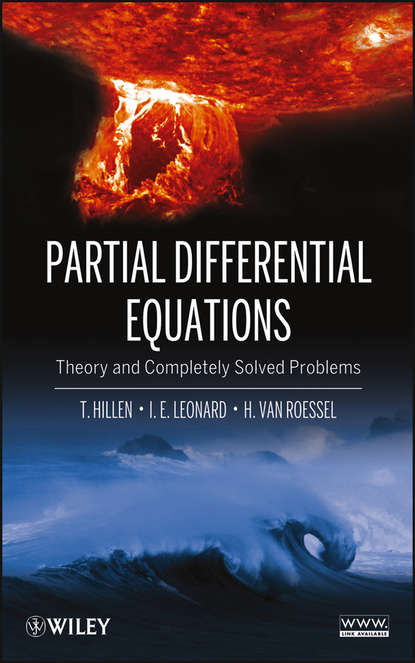Нашли ошибку

Перетащите или выберите файл# Partial Differential Equations. Theory and Completely Solved Problems

Автор
Год написания книги
2018
Uniquely provides fully solved problems for linear partial differential equations and boundary value problems Partial Differential Equations: Theory and Completely Solved Problems utilizes real-world physical models alongside essential theoretical concepts. With extensive examples, the book guides readers through the use of Partial Differential Equations (PDEs) for successfully solving and modeling phenomena in engineering, biology, and the applied sciences. The book focuses exclusively on linear PDEs and how they can be solved using the separation of variables technique. The authors begin by describing functions and their partial derivatives while also defining the concepts of elliptic, parabolic, and hyperbolic PDEs. Following an introduction to basic theory, subsequent chapters explore key topics including: • Classification of second-order linear PDEs • Derivation of heat, wave, and Laplace’s equations • Fourier series • Separation of variables • Sturm-Liouville theory • Fourier transforms Each chapter concludes with summaries that outline key concepts. Readers are provided the opportunity to test their comprehension of the presented material through numerous problems, ranked by their level of complexity, and a related website features supplemental data and resources. Extensively class-tested to ensure an accessible presentation, Partial Differential Equations is an excellent book for engineering, mathematics, and applied science courses on the topic at the upper-undergraduate and graduate levels.

## Читать онлайн

Авторизуйтесь чтобы можно было оставлять комментарии

## Отзывы

список сообщений пуст